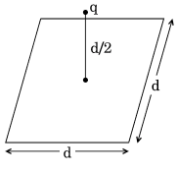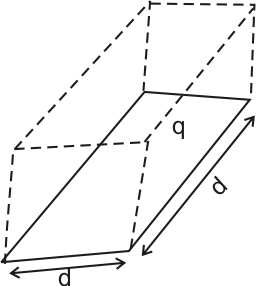# (a) Define electric flux. Is it a scalar or a vector quantity? A point charge q is at a distance of directly above the centre of a square of side d, as shown in the figure. Use Gauss’ law to obtain the expression for the electric flux through the square.(b) If the point charge is now moved to a distance ‘d’ from the centre of the square and the side of the square is doubled, explain how the electric flux will be affected.

a) Electric flux:- Electric flux through a given area is designed as the total number of electric lines of force passing normal to that area. ie it can be measure by taking the dot product of electric field  'E' and area 'ds' perpendicular to the field
That is the electric flux

electric flux is a scalar quantity
Construct a cube of 'd' and the charge 'q' is placed at the centre of cubeAccording to the Gauss’ theorem the electric flux
In the case of the cube, it is the total electric flux through all the six faces of the cube
Therefore flux through the one face of the cube is

b) if the charge is moved to a distance d from the centre of the square and the side of the square is double then the cube is constructed of side 2d. The charge is at the centre of the cube. Again flux through the square is times of total electric flux. The total flux does not  change so flux through the square will not change and it remains as

### Preparation Products

##### Knockout KCET 2021

An exhaustive E-learning program for the complete preparation of KCET exam..

₹ 4999/- ₹ 2999/-
##### Knockout KCET JEE Main 2021

It is an exhaustive preparation module made exclusively for cracking JEE & KCET.

₹ 27999/- ₹ 16999/-
##### Knockout NEET Sept 2020

An exhaustive E-learning program for the complete preparation of NEET..

₹ 15999/- ₹ 6999/-
##### Rank Booster NEET 2020

This course will help student to be better prepared and study in the right direction for NEET..

₹ 9999/- ₹ 4999/-